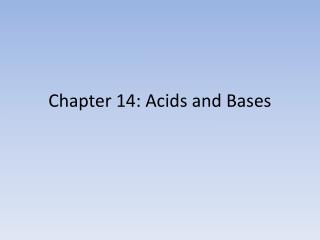DownloadDownload PresentationChapter 14: Acids and Bases

# Chapter 14: Acids and Bases

Télécharger la présentation## Chapter 14: Acids and Bases

- - - - - - - - - - - - - - - - - - - - - - - - - - - E N D - - - - - - - - - - - - - - - - - - - - - - - - - - -
##### Presentation Transcript

1. Chapter 14: Acids and Bases

2. Initial concepts of Acids and bases • First, acids were recognized as substances with a sour taste, but this was a dangerous way to identify. • The Arrhenius concept identified acids and bases as a result of the ions substances release in solution. • Arrhenius acids-release H+ ion in solution • Arrhenius bases-release OH- ion in solution

3. Initial concepts of Acids and bases • Circa 1900, Johannes Bronsted and Thomas Lowry suggested a more general definition • Bronsted-Lowry acid-proton(H+) donor. • Bronsted-Lowry base-proton acceptor. • For Example, when HCl (hydrochloric acid), each acid molecule donates a proton (H+) to a water molecule to form a hydronium Ion (H3O).

4. Acids HA(aq) + H2O(l) H3O+(aq) + A-(aq) • This is the generalized chemical equation when an acid is dissolved in water. • Take note that now the states of matter make a difference in what the equation is telling you. • HA-generalized formula for an acid. All acids have a “H” at the beginning of the formula, the “A” refers to a negatively charged atom or polyatomic ion. • Conjugate base (A-)-what remains after the acid loses the proton • Conjugate acid (H3O+ )-is formed when the proton is transferred to the base • Conjugate Acid-Base Pair-substances related to each other by donating and accepting a proton

5. Acids HCl(aq) + H2O(l) H3O+(aq) + Cl-(aq) • Even though we are following the Bronsted-Lowry model of acids, in this case we can identify all of the following: • Arrhenius acid: HCl-releases a proton which is accepted by H3O+ • Bronsted-lowryacid- HCl-dontates a proton to H3O+ • Lewis Acid-HCl-accepts an electron from the H2O to form H3O+

6. Acids HCl(aq) + H2O(l) H3O+(aq) + Cl-(aq) • Remember your definitions for conjugate acids and conjugate base. Lets identify them: • Conjugate base-what remains after the acid loses the proton-Cl- • Conjugate acid-is formed when the proton is transferred to the base- H3O+ • Conjugate acid is always positively charged (gain proton) • Conjugate base is always negative (lost proton) • Conjugate Acid-Base Pair-substances related to eachother by donating and accepting a proton

7. Important terms • Diprotic acids-able to donate two hydrogen ions (protons); mono-, tri-, poly; judged by chemical formula. • H2SO4 , HCl, HCN, • Most acids are oxyacids, in which the acidic protons is attached to a oxygen atom.

8. More examples • Identify the Lewis Acid, conjugate base, conjugate acid for each: • HC2H3O2(aq) H+(aq) + C2H3O2-(aq) • NH4+(aq)  H+(aq) + NH3(aq) • C6H5NH3+(aq)  H+(aq) + C6H5NH2(aq)

9. Acid Strength • Acid strength is defined by the equilibrium position of is dissociation • In other words, the stronger the acid, the stronger its ability to dissociate/ionize in water HA(aq) + H2O(l)↔ H3O+(aq) + A-(aq) • Strong acids are also strong electrolytes, the equilibrium lies to the right • Weak acids, the equilibrium lies to the left, weak electrolytes. • Measuring Ka

10. Acid Strength

11. Using/Calculating Ka • The ionization of a strong acid, such as HCl, will proceed to completion in reasonably dilute solutions. This process is written as follows. HCl(g) +H2O(l)→ H3O+(aq) + Cl-(aq) • This reaction goes 100% to the right; • NOT an equilibrium process, therefore there is not a equilibrium constant for this reaction.

12. Using/Calculating Ka • However, when weak acids and weak bases dissolve, they only partially ionize, resulting in an equilibrium between ionic and molecular forms. The following equation shows the equilibrium process that occurs when hydrogen fluoride, a weak acid, dissolves in water. HF(g)+ H2O(l)↔ H3O+(aq)+ F-(aq) • does not go 100% to the right; IS an equilibrium process • The equilibrium constants calculated for acid ionization reactions are called acid ionization constants and have the symbol Ka. The equilibrium constants calculated for base dissociation reactions are called base dissociation constants and have the symbol Kb.

13. General Plan for solving Kaand Kb

14. Example problem #1 • The hydronium ion concentration of a 0.500 M solution of HF at 25°C is found to be 0.0185 M. Calculate the ionization constant for HF at this temperature. • Remember that this is at equilibrium, so: • [HA] = [HF]initial -[H3O+]

15. Example problem #2 • At 25°C, a 0.025 M solution of formic acid, HCOOH, is found to have a hydronium ion concentration of 2.03 x 10-3 M. Calculate the ionization constant of formic acid.

16. Example #3 • At 25°C, the pH of a 0.315 M solution of nitrous acid, HNO2, is 1.93. Calculate the Kaof nitrous acid at this temperature. • Remember • pH=-log[HA] so: • [HA]=antilog(-pH) • So [H3O]=10-1.93

17. Example #4 • A 0.450 M ammonia solution has a pH of 11.45 at 25°C. Calculate the [H3O+] and [OH-] of the solution, and determine the base dissociation constant, Kb , of ammonia. • IMPORTANT!!! This is a base dissociation problem, and you are given a pH, not pOH. You must first convert to pOH